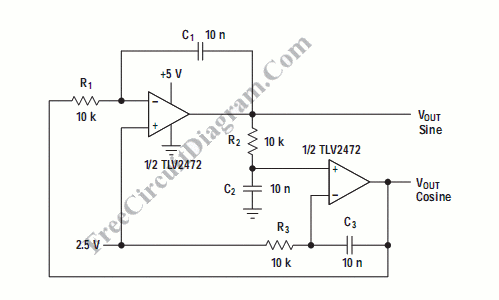# Quadrature OscillatorQuadrature oscillator generate two sine-wave signal, but one signal is shifted 90 degree from the other signal. One output that lead the other called cosine signal, and the other is called sine. Here is the schematic diagram of the circuit:This circuit is another type of pahase shift oscillator, with three phase shifter sections. Each section is configured to produce 90 degree pahase shift. With R1C1 = R2C2 =R3C3, the frequency f would be equal to 1/2[pi]RC.  When tested, this circuit produces 1.65 kH  rather than the calculated 1.59 kHz, as the components value might variate within their tolerance. [Schematic diagram source: Analog Application Journal, August 2000]